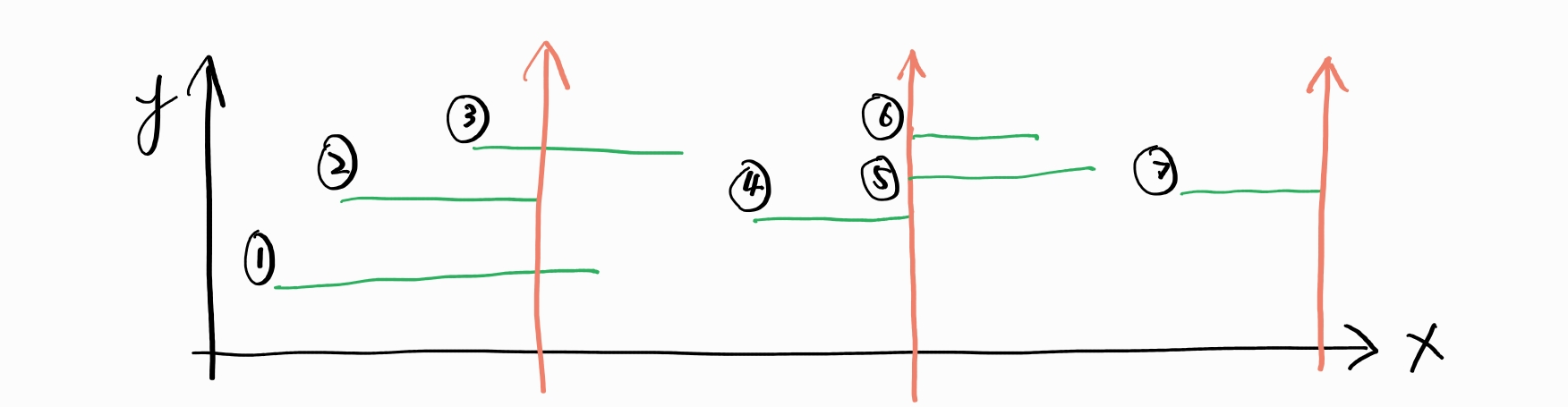## 452 爆破气球的最小箭数

There are some spherical balloons taped onto a flat wall that represents the XY-plane. The balloons are represented as a 2D integer array points where points[i] = [xstart, xend] denotes a balloon whose horizontal diameter stretches between xstart and xend. You do not know the exact y-coordinates of the balloons.

Arrows can be shot up directly vertically (in the positive y-direction) from different points along the x-axis. A balloon with xstart and xend is burst by an arrow shot at x if xstart <= x <= xend. There is no limit to the number of arrows that can be shot. A shot arrow keeps traveling up infinitely, bursting any balloons in its path.

Given the array points, return the minimum number of arrows that must be shot to burst all balloons.1 2 3 4 5 6 7 8 9 10 11 12 13 14 15 16 17 18  class Solution { public int findMinArrowShots(int[][] points) { if (points.length == 0) return 0; Arrays.sort(points, Comparator.comparingInt(o -> o)); int res = 0; int nextBinary = Integer.MAX_VALUE; for (int[] point : points) { if (point > nextBinary) { res++; nextBinary = point; continue; } nextBinary = Math.min(nextBinary, point); } return res + 1; // 最后一个气球要射一次 } } 

## 435 无重叠区间

Given an array of intervals intervals where intervals[i] = [starti, endi], return the minimum number of intervals you need to remove to make the rest of the intervals non-overlapping.

^ Y
|   ---------   ------------
|          ------
-------------------------------> X


  1 2 3 4 5 6 7 8 9 10 11 12 13 14 15  class Solution { public int eraseOverlapIntervals(int[][] intervals) { Arrays.sort(intervals, Comparator.comparingInt(o -> o)); int count = 0; int lastRight = Integer.MIN_VALUE; for (int[] interval : intervals) { if (interval >= lastRight) { count++; lastRight = interval; } lastRight = Math.min(lastRight, interval); } return intervals.length - count; } } 

## 135 分发糖果

There are n children standing in a line. Each child is assigned a rating value given in the integer array ratings.

You are giving candies to these children subjected to the following requirements:

• Each child must have at least one candy.
• Children with a higher rating get more candies than their neighbors.

Return the minimum number of candies you need to have to distribute the candies to the children.

  1 2 3 4 5 6 7 8 9 10 11 12 13 14 15 16 17 18 19 20 21 22  class Solution { public int candy(int[] ratings) { if (ratings.length == 0) return 0; int[] candies = new int[ratings.length]; // 只考虑左边 candies = 1; for (int i = 1; i < candies.length; i++) { candies[i] = ratings[i] > ratings[i - 1] ? candies[i - 1] + 1 : 1; } // 只考虑右边，取大 for (int i = candies.length - 2; i >= 0; i--) { int newValue = ratings[i] > ratings[i + 1] ? candies[i + 1] + 1 : 1; candies[i] = Math.max(candies[i], newValue); } // 计算答案 int res = 0; for (int candy : candies) { res += candy; } return res; } } 

## 714 买卖股票

You are given an array prices where prices[i] is the price of a given stock on the ith day, and an integer fee representing a transaction fee.

Find the maximum profit you can achieve. You may complete as many transactions as you like, but you need to pay the transaction fee for each transaction.

Note: You may not engage in multiple transactions simultaneously (i.e., you must sell the stock before you buy again).

  1 2 3 4 5 6 7 8 9 10 11 12 13 14 15  class Solution { public int maxProfit(in t[] prices, int fee) { int minPrice = prices; int result = 0; for (int i = 1; i < prices.length; i++) { if (prices[i] < minPrice) minPrice = prices[i]; // 低价买入 if (prices[i] - minPrice > fee) { result += prices[i] - minPrice - fee; minPrice = prices[i] - fee; // 下一次如果连涨，就补偿手续费；如果不连涨，minPrice 会自动重置 } } return result; } }